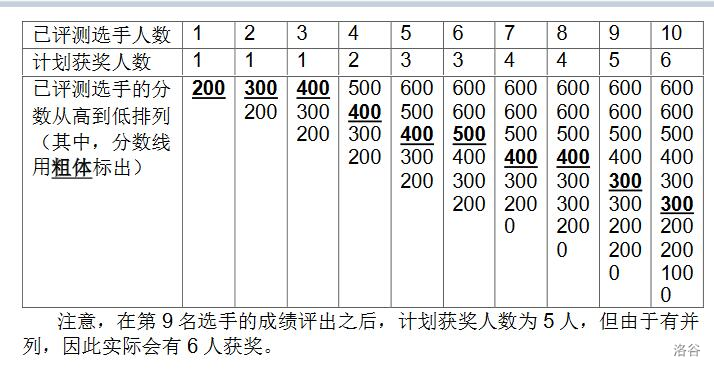## 题目描述

NOI2130 即将举行。为了增加观赏性，CCF 决定逐一评出每个选手的成绩，并直播即时的获奖分数线。本次竞赛的获奖率为 w%，即当前排名前 w% 的选手的最低成绩就是即时的分数线。

## 输入输出样例

10 60
200 300 400 500 600 600 0 300 200 100


200 300 400 400 400 500 400 400 300 300


10 30
100 100 600 100 100 100 100 100 100 100


100 100 600 600 600 600 100 100 100 100


## 说明/提示

### 样例 1 解释### 数据规模与约定

$1 \sim 3$ $10$
$4 \sim 6$ $500$
$7 \sim 10$ $2000$
$11 \sim 17$ $10^4$
$18 \sim 20$ $10^5$

## 题目分析

1. 每次排名人数 $k_i$ 不是一成不变的，是会变化。
2. 每次输入的分数会导致，排名会发生变化。
3. 数据规模太大。

## 代码实现

#include <iostream>
#include <cstdio>
using namespace std;
const int N=1e5+5;
int sc;//sc[x]=x出现的次数
int main(){
int n,w,x;
cin>>n>>w;
for(int i=1;i<=n;i++){
cin>>x;
sc[x]++;//统计x出现的次数
int num=max(1,i*w/100);//计算排名，注意最少选一个人
int sum=0;//总人数
for(int j=600;j>=0;j--){//遍历最高分到最低分
sum+=sc[j];//累加该分段的人数
if(sum>=num){//达到排名
cout<<j<<" ";//输出当前分数
break;//结束
}
}
}
return 0;
}